NCERT Solutions for Class 7 Maths Chapter 3 Data Handling Ex 3.1 are part of NCERT Solutions for Class 7 Maths. Here we have given NCERT Solutions for Class 7 Maths Chapter 3 Data Handling Ex 3.1.

 Board CBSE Textbook NCERT Class Class 7 Subject Maths Chapter Chapter 3 Chapter Name Data Handling Exercise Ex 3.1 Number of Questions Solved 9 Category NCERT Solutions

## NCERT Solutions for Class 7 Maths Chapter 3 Data Handling Ex 3.1

Question 1.
Find the range of heights of any ten students of your class.
Solution:
Let the heights (in cm) of 10 students in the class be 150, 152, 151, 148, 149, 149, 150, 152, 153, 146.
Arranging the heights in ascending order, we have 146, 148, 149, 149, 150, 150, 151, 152, 152, 153.
Range of height of students = 153 – 146 = 7

Question 2.
Organize the following marks in a class assessment, in a tabular form.
4, 6, 7, 5, 3, 5, 4, 5, 2, 6, 2, 5, 1, 9, 6, 5, 8, 4, 6, 7
(i) Which number is the highest?
(ii) Which number is the lowest?
(iii) What is the range of the data?
(iv) Find the arithmetic mean.
Solution:(i) Highest number is 9.
(ii) Lowest number is 1.
(iii) Range of the data = Highest observation – Lowest observation
= 9 – 1
= 8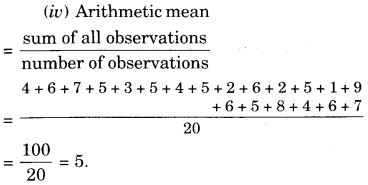Question 3.
Find the mean of the first five whole numbers.
Solution:
The first 5 whole numbers are 0, 1, 2, 3, and 4.
Their arithmetic mean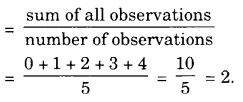Question 4.
A cricketer scores the following runs in eight innings:
58, 76, 40, 35, 46, 45, 0, 100.
Find the mean score.
Solution: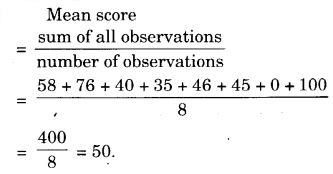Question 5.
Following table shows the points of each player scored in four games: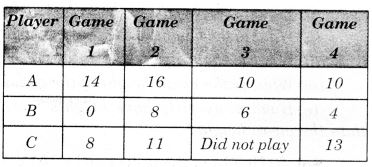1. Find the mean to determine A’s average number of points scored per game.
2. To find the mean number of points per game for C, would you divide the total points by 3 or by 4? Why?
3. B played in all four games. How would you find the mean?
4. Who is the best performer?

Solution: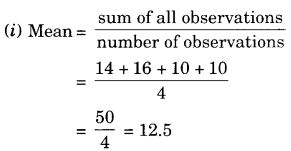So, A’s average number of points scored per game is 12.5.
(ii) To find the mean number of points per game for C, we shall divide the total points by 3 because the number of games under consideration is 4 but ‘C’ did not play game 3.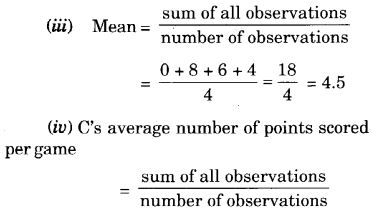Question 6.
The marks (out of 100) obtained by a group of students in a science test are 85, 76, 90, 85, 39, 48, 56, 95, 81 and 75. Find the:

1. Highest and the lowest marks obtained by the students.
2. Range of the marks obtained.
3. Mean marks obtained by the group.

Solution:

1. Highest marks obtained by the students = 95
Lowest marks obtained by the students = 39
2. Range of the marks obtained = Highest marks – Lowest marks = 95 – 39 = 56
3. Mean marks obtained by the group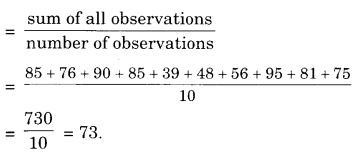Question 7.
The enrolment of a school during six consecutive years was as follows:
1555, 1670, 1750, 2013, 2540, 2820.
Find the mean enrolment of the school for this period.
Solution:
Mean enrolment of the school for this period.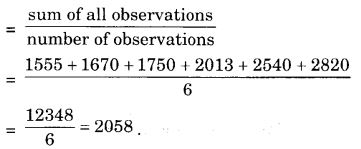Question 8.
The rainfall (in mm) in a city on 7 days of a certain week was recorded as follows:(i) Find the range of the rainfall in the above data.
(ii) Find the mean rainfall for the week.
(iii) On how many days was the rainfall less than the mean rainfall?
Solution:
(i) Range of the rainfall = Highest rainfall – Lowest rainfall = 20.5 mm – 0.0 mm = 20.5 mm
(ii) Mean rainfall for the week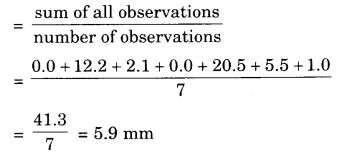(iii) The rainfall was less than the mean rainfall on 5 days.

Question 9.
The heights of 10 girls were measured in cm and the results are as follows:
135, 150, 139, 128, 151, 132, 146, 149, 143, 141.
(i) What is the height of the tallest girl?
(i) What is the height of the shortest girl?
(iii) What is the range of the data?
(iv) What is the mean height of the girls?
(v) How many girls have heights more than the mean height?

Solution:
(i) Height of the tallest girl = 151 cm
(ii) Height of the shortest girl = 128 cm
(iii) Range of the data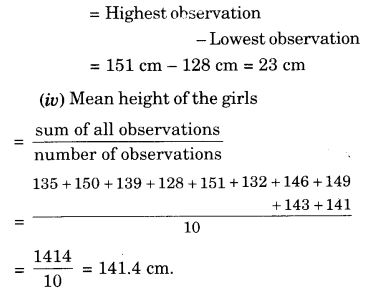(v) 5 girls have heights more than the mean height.

We hope the NCERT Solutions for Class 7 Maths Chapter 3 Data Handling Ex 3.1 helps you. If you have any query regarding NCERT Solutions for Class 7 Maths Chapter 3 Data Handling Ex 3.1, drop a comment below and we will get back to you at the earliest.